# RD Sharma Solutions - Chapter 19 - Visualising Shapes (Part - 2), Class 8, Maths Notes | Study RD Sharma Solutions for Class 8 Mathematics - Class 8

## Class 8: RD Sharma Solutions - Chapter 19 - Visualising Shapes (Part - 2), Class 8, Maths Notes | Study RD Sharma Solutions for Class 8 Mathematics - Class 8

The document RD Sharma Solutions - Chapter 19 - Visualising Shapes (Part - 2), Class 8, Maths Notes | Study RD Sharma Solutions for Class 8 Mathematics - Class 8 is a part of the Class 8 Course RD Sharma Solutions for Class 8 Mathematics.
All you need of Class 8 at this link: Class 8

PAGE NO 19.12:

Question 1:

Which among the following are nets for a cube?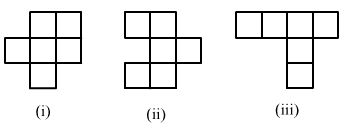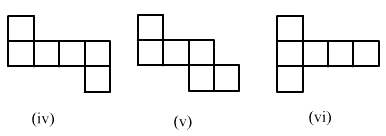To create a cube, we need six equal faces that enclose a closed box.

In the given figure, only (iv) , (v)  and (vi) are such nets that enclose a box when we fold each face from the edge.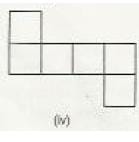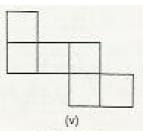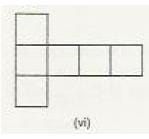PAGE NO 19.13:

Question 2:

Name the polyhedron that can be made by folding each net: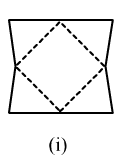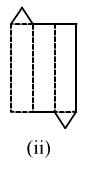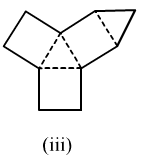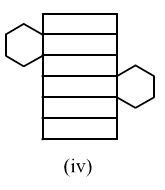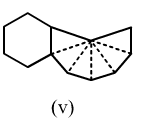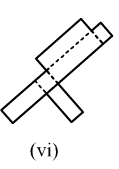(i) If we fold the given figure from the edges, we'll get a pyramid with a square base.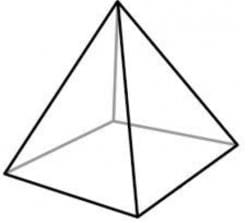(ii) If we fold the given polyhedron from the edges, we'll get a triangular prism.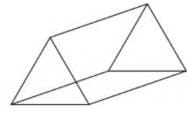(iii) If we fold the given polyhedron from the edges, we'll get a triangular prism.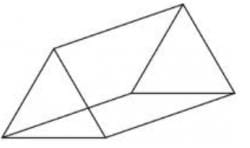(iv) If we fold the given polyhedron from the edges, we'll get a hexagonal prism.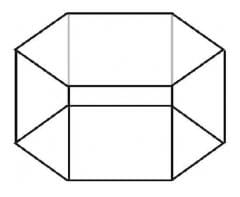(v) If we fold the given net from the edges, we'll get a hexagonal pyramid.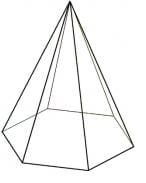(vi) If we fold the given net from the edges, we'll get a cuboid.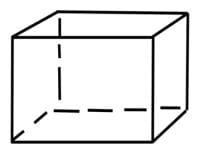Question 3:

Dice are cubes where the numbers on the opposite faces must total 7. Which of the following are dice?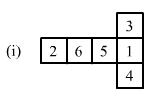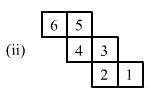Among the given figures, only figure (i)  is a dice.

This is because if we fold the given net from the edges, we'll get a cube in which the sum of the opposite faces is 7.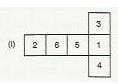Question 4:

Draw nets for each of the following polyhedrons: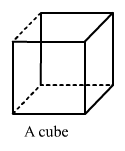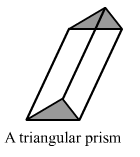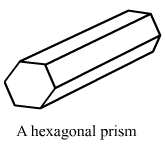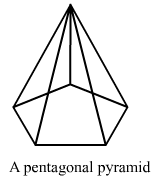(i)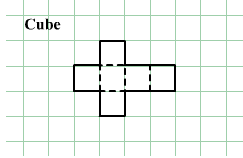(ii)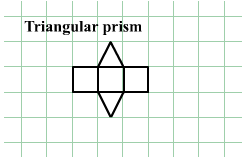(iii)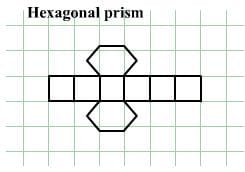(iv)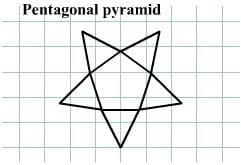PAGE NO 19.14:

Question 5:

Match the following figures: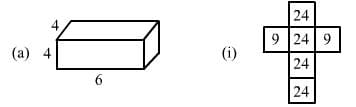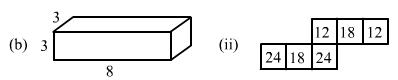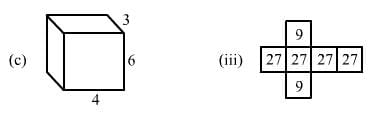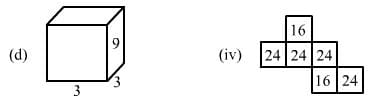(a) The given figure is a cuboid with sides 4, 4 and 6 units.

Area of a rectangle  = length × width

∴ Area of the rectangular face sith sides 4 and 4  = 4 × 4 = 16

And, area of the other face with sides 4 and 6  = 4 × 6 = 24

Thus, the net for the given figure will have four faces with area 24 and two faces with area 16.

Observe net (iv)  satisfies this.Thus, the net of figure (a)  is net (iv) .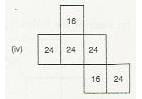(b) The given figure is a cuboid with sides 3, 3 and 8.

Area of a rectangle  = length × width

∴ Area of the rectangular face sith sides 3 and 3  = 3 × 3 = 9

And the area of the other face with sides 3 and 8  = 3 × 8 = 24

Thus, the net for given figure will have four faces with area 24 and two faces with area 9.

Observe that net (i)  satisfies this.Thus, the net of figure (b)  is net (i) .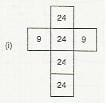(c) The given figure is a cuboid with sides 3, 4 and 6.

Area of a rectangle = length × width

∴ Area of the rectangular face sith sides 3 and 4  = 3 × 4 = 12,

Area of the rectangular face with sides 4 and 6  = 4 × 6 = 24

And, area of the other face with sides 3 and 6  = 3 × 6 = 18

Thus, the net for given figure will have two faces with area 24, two faces with area 18 and two faces with area 12.

Observe that net (ii)  satisfies this.Thus, the net of figure (c)  is net (ii) .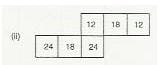(d) The given figure is a cuboid with sides 3, 3 and 9.Area of a rectangle  = length × width

Area of the rectangular face with sides 3 and 3  = 3 × 3 = 9,

And, area of the other face with sides 3 and 9  = 3 × 9 = 27

Thus, the net for given figure will have four faces with area 27 and two faces with area 9.

Observe that net (iii)  satisfies this.

Thus, the net of figure (d)  is net (iii) .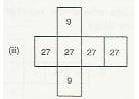The document RD Sharma Solutions - Chapter 19 - Visualising Shapes (Part - 2), Class 8, Maths Notes | Study RD Sharma Solutions for Class 8 Mathematics - Class 8 is a part of the Class 8 Course RD Sharma Solutions for Class 8 Mathematics.
All you need of Class 8 at this link: Class 8Use Code STAYHOME200 and get INR 200 additional OFF

## RD Sharma Solutions for Class 8 Mathematics

88 docs

Track your progress, build streaks, highlight & save important lessons and more!

,

,

,

,

,

,

,

,

,

,

,

,

,

,

,

,

,

,

,

,

,

,

,

,

,

,

,

;